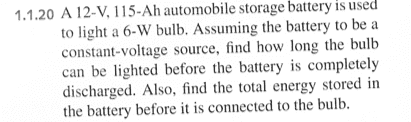# Circuits: Total Energy in a Battery Before Connecting to Bulb

In summary, the first part of the homework is solved using dimensional analysis to find that I=0.5A. The second part is not sure how to get the total energy stored in the battery before it is connected.f

## Homework StatementP = IV

## The Attempt at a Solution

Part 1
For the first part I solved P = IV for the current flowing through the bulb to find that I = 0.5 A. I then divided 115 Ah by 0.5 A to get 230 hours. Now, I don't really understand what I did here. I just used dimensional analysis to come up with the answer. If anyone can suggest a more mathematical approach or physical approach, that would be nice.

Part 2

I am not really sure how to get the total energy stored in the battery before it is connected. This number is independent of the light bulb yes? My professor suggested that it was not, but I may have confused what he said.

I know that battery offers a potential of V(t) = 12 volts and a total charge of qtot = (115*3600) C.

I am not sure where to go with this information?

The first part is fine; might be a little nicer if you used
Power = Energy/time or Energy = Power * time
You certainly need that formula for the part b.
You are quite right, the energy in the battery should be independent of how it may be used.

Hello Again Delphi!

Well, I want to use $P = dE/dt = IV$ but I don't really have an expression for the current or the power. So I am not really sure how to use that formula in Part 1 or Part 2? I am not given the power either. I am given the potential V and the Charge.

EDIT: Ok, I had the power of the bulb for part 1 but not part 2 since the bulb is not a factor.

Last edited:
In part 2, just use E = P*t = I*V*t = 115*12*3600, assuming a 115Ah rating means it can put out 115 A for one hour.
If you want to use the charge, you could use the definition of potential as the energy per charge, so E = q*V.

In part 2, just use E = P*t = I*V*t = 115*12*3600, assuming a 115Ah rating means it can put out 115 A for one hour.
If you want to use the charge, you could use the definition of potential as the energy per charge, so E = q*V.

I like it! Thanks for pointing that out. Forgot by definition Potential = Energy/unit chargeMost welcome. Good luck with the next one!

Most welcome. Good luck with the next one!

Ha! ThanksI'm doing a combined MS/BS degree in mechanical engineering. I am in my very last semester and I have 3 grad mechanical classes and this second year EE stinker class that I am required to takeI like the lab though, seems pretty useful!